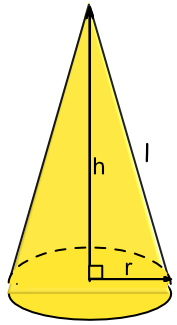Surface Area of a Cone

## Surface Area of a Cone

There are two formulae available for the surface area of a cone. The difference between them is based on whether the slope height or the vertical height is available.

A = pir^2 + pirl if the sloping height is known.

A = pir(r + sqrt(h^2 + r^2)) if the vertical height is known;

The second formula is a variation on the first and incorporates Pythagoras Theorem into the formulae.

Both formulae include the surface area of the base of the cone.## Example 1

A cone has a radius of 6cm and a vertical height of 12cm. Give the total surface area of the cone to 1 decimal place.

 For a Cone Area = pir(r+sqrt(h^2 + r^2)) Substitute A = pi(6)((6)+sqrt((12)^2+(6)^2) = pi(6)((6)+sqrt(180) = 365.99

Use A = pir^2 + pirl, but only the sloping part of the formula is needed as pir^2 refers to the base part.